×#### Thank you for registering.

One of our academic counsellors will contact you within 1 working day.

Click to Chat

1800-1023-196

+91-120-4616500

CART 0

• 0

MY CART (5)

Use Coupon: CART20 and get 20% off on all online Study Material

ITEM
DETAILS
MRP
DISCOUNT
FINAL PRICE
Total Price: Rs.

There are no items in this cart.
Continue Shopping```Formation of Differential EquationsWe  know  y2 = 4ax  is a  parabola  whose  vertex is at origin and axis as the x-axis .If a  is a parameter, it  will represent a family of parabola with the  vertex  at (0,  0) and  axis as  y = 0 .

Differentiating y2 = 4ax        . .  (1)

2y dy/dx = 4a     . .  (2)

From (1) and (2), y2 = 2yxdy/ dx & y = 2xdy /dx

This is a differential equation  for all  the  members  of the  family   and  it does  not  contain any  parameter  ( arbitrary constant).

(1) The differential equation of a family of curves of one parameter is a differential equation of the first order, obtained by eliminating the parameter by differentiation.

(2) The differential equation of a family of curves of two parameters is a differential equation of the second order, obtained by eliminating the parameter by differentiating the algebraic equation twice. Similar procedure is used to find differential equation of a family of curves of three or more parameter.

Example: Find the differential equation of the family of curves y = Aex + Be3x for different values of A and B.

Solution:           y = A ex + Be3x                                 . . . . (1)

y1 = Aex + 3Be3x                                . . .  (2)   (y1 = dy/dx)

y2 = Aex + 9B3x                                 . . .  (3) (y2 = d2y/dx2)

Eliminating A and B from the above three, we get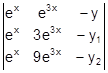= 0   so, ex e3x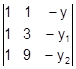= 0
3y + 4y1 – y2 = 0 or 3y + 4dy/dx – d2y/ dx2 =0.

Solutions of Differential Equation

The general solution of a differential equation is the relation in the variables x, y obtained by integrating (removing derivatives) where the relation contains as many arbitrary constants as the order of the equation. The general solution of a differential equation of the first order contains one arbitrary constant while that of the second order contains two arbitrary constants. In the general solution, if particular values of the arbitrary constant are put, we get a particular solution which will give one member of the family of curves.

To solve differential equation of the first order and the first degree:

Simple standard forms of differential equations of the first order and first degree are as follows:

(i)Variable Separable

Form f(x) dx + f(y) dy = 0

Method: Integrate it i.e., find ∫f(x) dx + ∫f(y) dy = c

Example: Solve dy/dx= ex y.

Solution:Given  dy/dx= ex y.

Separating the terms of x and y we get, dy/y =ex dx

Integrating, we get ln y – ex =c

Example: Solve dy/ dx = x2

Solution: Separating the terms of x on one side and of y on the other, we get

dy = x2 dx

Integrating both sides, y= x3/3 + c, where c is an arbitrary constant.

Watch this Video for more reference

(ii)Reducible into Variable Separable:

Method: Sometimes differential equation of first order cannot be solved directly by variable separation but by some substitution we can reduce it to a differential equation with separable variables.

A differential equation of the dy/ dx = f (ax+by+c) is solved by writing ax + by + c = t

Example:Solve (x – y)2 dy/dx = 1.

Solution:Put z = x –y so dz /dx = 1- dy/dx

Hence, dy/ dx = 1- dz/ dx

Hnce, substituting these values in the given equation, z2(1-dz/dx) = 1

Now z2(1-dz/dx) = 1and so z2 dz/dx = z2 -1.

So, dx = z2 dz/ z2 -1, which is in the form of variable separable

Now integrating, we get x = z + ½.ln (z-1/ z+1) +c

Solution is x =(x – y) + ½ ln (x-y-1/ x-y+1) +1.

Example: cos (x+y+1) dx- dy = 0

Solution: Rearranging the terms it can easily be reduced to variable separable.

So, dy / dx= cos (x+y+1)

If we substitute t = x+y+1, we get

dt/ dx = 1+ dy/dx

So, dy/dx = (dt/dx)-1.

Hence the equation becomes, (dt / dx) -1 = cos t

So, dt / dx = 1+ cos t

Now taking the terms of t on one side and of x on the other

dt / (1+cos t) = dx ……(1)

For integrating (1+cos t), first we write it as 2 cos 2t/2 using the formula cos 2x = 2 cos2x-1.

Now integrating dt/ 2 cos2 (t/2) i.e. ½ sec 2 (t/2) gives tan t/2. So, using this in equation (1) we get

tan t/2 – x = c

Hence, tan [(x+y+1)/2] –x = c.

You may also consult the Sample Papers to get an idea about the type of questions asked.

(iii)Homogeneous Equation

When dy/dx is equal to a fraction whose numerator and the denominator both are homogeneous functions of x and y of the same degree then the differential equation is said to be homogeneous equation.

i.e.  When dy/dx = f(x,y), where   f (km, ky) = f(x, y) then this differential equation is said to be homogeneous differential equation.

Method:  Put   y = vx

Example: dy/ dx = (x+y) / x

Solution: Clearly the given equation is homogeneous.

So putting y = vx, we get

dy/ dx = v+ xdv/ dx

Hence, the given equation becomes

v+ xdv/ dx = (x+vx) /x

v+ xdv/ dx = 1+v

xdv/ dx = 1.

Hence, again separating the terms of x and v, we get

dv = dx/ x

Integrating, v= ln x + c

Hnece, y/ x = ln x +c

y= x ln x + cx

Example: Solve dy/dx = x2 +y2 / 2xy.

Solution: dy/dx = x2 +y2 / 2xy (homogeneous). Put y = vx

x2 + v2x2 / 2xvx = v+x dv/dx

dx/x = 2v / 1-v2 dv

Integrate

C + lnx = - ln(1 –v2)

lnkx + ln(1 –v2) =0

kx (1- v2) = 1  k(x2 – y2) = x .

(iv) Non-homogeneous Differential Equation

Form dy/ dx = (a1x +b1y +c1) / (a2x +b2y + c2)

Method: If a1/a2 ≠ b1 /b2 ,  put  x = X + h ,  y = Y + k such that a1h +b1k + c1 =  0,  a2h + b2k +c2 = 0 . In this way the equation becomes homogeneous in X, Y. then use the method for homogeneous equation.

If a1/a2 = b1 /b2, Put a1x+b1y = v or a2x+b2y = v. The equation is in the form of variable separable in x and v.

Example 5:  dy/ dx = (x-y+3) / (2x – 2y +5).

Solution:  Here a1/a2 = b1 /b2 = 1/2

Hence we put x- y = v

1-dy/dx = dv/dx so dy/dx = 1- dv/dx

1-dv/dx = v+3 / 2v +5

So, (2v+5)/( v+2) dv = dx

[2+ 1/ (v+2) ]dv=dx

Integrate

2v + ln (v +2) = x + C, Put the value of v

x – 2y + ln (x – y +2) = C

(v)Linear Equation

Form dy/dx +P(x)y = Q(x), where P(x)  and  Q(x) are  functions  of x

Method: Multiplying the equation by e ∫ P(x) dx, called integrating factor. Then the

equation becomesIntegrating(vi) Reducible into Linear Equation

Form R(y)dy/dx + P(x) S(y) = Q(x) , such  that d[S(y)]/ dy = R(y)

Method:  Put S(y) =z then dz/dx = d[S(y)] / dx = R(y) dy/dx

The equation becomes dz/dx +p(x) z = Q(x), which is in the linear form.

Example: dy/dx + y/ (1+x2) = e tan-1x / (1+x2).

Solution: dy/dx + y/ (1+x2) = e tan-1x / (1+x2).

Multiplying both sides by I.F. and integrating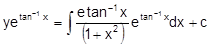Put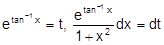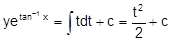2 y(vii)Exact Differential Equations

Mdx + Ndy = 0, where M and N are functions of x and y. If  ∂M/∂y =∂N/∂x, then the  equation  is exact  and  its  solution is  given  by  ∫ Mdx + ∫ N dy  = c

To find the solution of an exact differential equation Mdx + N dy = 0, integrate ∫ Mdx  as if y were constant. Also integrate the terms of N that do not contain x w.r.t y. Equate the sum of these integrals to a constant.

Example -7:  (x2 –ay)dx + (y2 –ax)dy = 0.

Solution: Here M = x2 –ay

N = y2 –ax

∂M/∂y =-a

∂N/∂x =-a

Since, ∂M/∂y= ∂N/∂x equation is exact

Solution is ∫ (x2-ay) dx + ∫y2 dy = c

x3/3 –ayx + y3/3 = c.

or x3 –3axy + y3 = 3c.

Integrating Factor:

A factor, which when multiplied to a non-exact, differential equation makes it exact, is known as integrating factor e.g. the non-exact equation y dx –x dy = 0 can be made exact on multiplying by the factor 1/y2. Hence 1/y2 is the integrating factor for this equation.

Notes:

In general such a factor exists but except in certain special cases, it is likely to be difficult to determine.

The number of integrating factor for equation M dx + N dy = 0 is infinite.

Some Useful Results:

d(xy) = xdy + ydx

d(y/x) = (xdy-ydx)/ x2

d(x/y) = (ydx-xdy) / y2

d [ln(y/x)] = (xdy-ydx)/ xy

d [tan -1(y/x)] = (xdy-ydx)/ x2+y2

d [tan -1(x/y)] = (ydx-xdy)/ x2+y2

d [sin -1(xy)] = (xdy+ydx)/ √1-x2y2

Example:Solve  x dy – y dx  = √x2+y2dx.

Solution: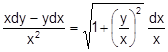ÞÞ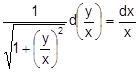Integrating

Þ  ln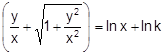Þ ln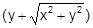= 2 ln x + ln k

y  +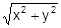= kx2.

(viii)Linear Differential Equation with constant coefficient

Differential equation of the form a0 dny/dxn + a1dn-1y/dxn-1  + ….+ an y = 0, ai ∈ R for  i =  0, 1 , 2, 3, . . . , n  is  called   a linear  differential equation   with  constant  coefficients.

In order to solve this differential equation, take the auxiliary equation as a0Dn + a1Dn-1 +…+ an =0

Find the roots of this equation and then solution of the given differential equation will be as given in the following table

Roots  of the auxiliary equation

Corresponding complementary function

1

One  real root  a1

C1eα1x

2.

Two real and  differential root a1 and a2

C1eα1x + C2eα2x

3.

Two  real  and  equal roots  a1 and a2

(C1 + C2x) eα1x

4.

Three real  and  equal roots a1, a2, a3

(C1 + C­2x + C3x2 ) eα1x

5.

One  pair  of  imaginary  roots  a±  ib

(C1  cosbx + C2 sinbx) eαx

6.

Two Pair  of equal  imaginary roots
a±  ib and a± ib

[ (C1 + C2x) cosb  + (C1 + C2x)sinb] eαx

Example: Solve d2y/dx2 -3dy/dx +2 = 0.

Solution: Its auxiliary equation is D2 –3D + 2 = 0 so D = 1, D = 2

Hence its solution is y = C1ex + C2e2x

So far only linear differential equation with constant coefficients of form

a0 dny/dxn + a1dn-1y/dxn-1  + ….+ an y = 0,  ai ∈R

for i = 0, 1, 2, …., n were considered. Now we consider the following form

a0 dny/dxn + a1dn-1y/dxn-1  + ….+ an y= X

where X is either constant or functions of x alone.

Theorem:

If y = f1(x) is the general solution of a0 dny/dxn + a1dn-1y/dxn-1 + …. + an y= 0 and  f2(x)  is a solution of

a0 dny/dxn + a1dn-1y/dxn-1  + ….+ an y= X

Then y = f1(x) + f2(x) is the general solution of a0 dny/dxn + a1dn-1y/dxn-1  + ….+ an y= X

Expression f1(x)  is known as complementary function and the expression f2(x) is known as particular integral. These are denotes by C.F.  and P.I. respectively.

The nth derivative of y will be denoted Dny where D stands for d/dx and n denotes the order of derivative.

If we take Differential Equation:

dny/dxn + P1dn-1y/dxn-1 + …. + Pny= X

then we can write this differential equation in a symbolic form as

Dny + P1Dn– 1y + P2Dn– 2y + ….+ Pny = X

(Dn + P1Dn– 1 + P2Dn– 2 + ….+ Pn)y = X

The operator Dn + P1Dn– 1 + P2Dn– 2 + …. + Pn­ is denoted by f (D) so that the equation takes the form f (D) y = X

y = 1 / f(D) . X

To read more, Buy study materials of Differential Equations comprising study notes, revision notes, video lectures, previous year solved questions etc. Also browse for more study materials on Mathematics here.
```### Course Features

• 731 Video Lectures
• Revision Notes
• Previous Year Papers
• Mind Map
• Study Planner
• NCERT Solutions
• Discussion Forum
• Test paper with Video Solution